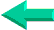### This is an excerpt from Pierre Leclerc (www.excel-vba.com)

SUMPRODUCT  ¦  INDEX/MATCH  ¦  SUBTOTAL  ¦  ISERROR  ¦  HLOOKUP  ¦  LOOKUP  ¦  VLOOKUP
AVERAGEIF  ¦  AVERAGEIFS ¦  COUNTIF  ¦  COUNTIFS  ¦  SUMIF  ¦  SUMIFS  ¦  IFERRORBack home

The 2 Most Important

Excel Functions AND
Formulas

SUMPRODUCT

AND

INDEX/MATCH

## Lesson 17: Microsoft Excel Signs

Here are the 21  signs that you can use to develop Excel formulas.

Notice that when you start copy/pasting long formulas (using SUMPRODUCT or INDEX/MATCH) you will start using the dollar sign (\$). Here is a very useful tip: to add \$ to addresses (making them relative or absolute) click on the address within the address bar (above the Excel grid) and use the F4 key at the top of your keyboard once, twice, three or four times.

Notice that the + and * signs are essential  when you start using the most important, useful and powerful function in Excel: SUMPRODUCT

 Signs What it Does = Equals (all formulas begin with an equal sign.) ( Open parenthesis ) Close parenthesis , Separating arguments : From A1 to A23   A1:A23 + Plus. *** Also:  used to submit more than one argument as criteria within a SUMPRODUCT formula. - Minus * Multiplies.   *** Also:  used to separate arguments in SUMPRODUCT formulas / Divides < Smaller than: used mostly within IF formulas > Greater than: used mostly within IF formulas " " What is within the quotes is text & Working with text, assembling strings (chains of characters), concatenation (Space) Separating arguments (Metric system) \$ Absolute/Relative References ^ Returns the result of a number raised to a power ' Transforms any content into text [ Surrounds the name and path of another workbook to which refers a formula. ] { Surrounds and identifies array formulas that are entered with SHIFT/CTRL/ENTER } Surrounds and identifies array formulas that are entered with SHIFT/CTRL/ENTER

Examples

=A will result in the error message #Name?  because Excel does not know a function by the name of A.

=" A" will result  in A because you are saying with the quotation marks  that you want this cell to carry the character A.

=A1 will result in the value of cell A1 be it a number, a date or a string of character.

=3 will result in the number 3

=A1+A2+A3 will result in the sum of cells A1, A2 and A3. You can also use the SUM function =SUM(A1:A3) the colon meaning from/to.

=10/A1 will result in 10 divided by the value of cell A1. If cell A1 is empty or contains zero you end up with the error message #DIV/0!.

=IF(A1> 90," A" ," B" ) in plain English this formula says if the value of cell A1 is greater than 90 then the value of the cell in which resides this formula should be the letter A otherwise it should be the letter B. Notice the commas separating the three arguments of this IF formula. IF(condition, value if condition is true, value if condition is false)

=IF(A1< > 100,0,100) in plain English this formula says if the value of cell A1 is different  than 90 then the value of the cell in which resides this formula should be 0  otherwise it should be 100

=IF(A1< =100,0,100)in plain English this formula says if the value of cell A1 is smaller then or equal to 100  then the value of the cell in which resides this formula should be 0  otherwise it should be 100

If in cell A1 you have " Peter" and in cell A2 you have " Clark" the formula =A1 & A2 in A3 will result in " PeterClark" . If you want a space between the first name and surname you will use the formula =A1 & " " & A2 telling Excel to insert a space (Space between double quotes) between the values of cell A1 and cell A2.

You must learn to master the use of the dollar sign (\$) if you want to start developing long and complex formulas that you would want to copy/paste. To insert \$ signs within an address select it in the formula bar and click on the F4 key once, twice, three or four times as needed.

If in cell A1 you have the formula =B6 it will become =B7 when you copy/paste it in cell A2 and it will become =C6 if you copy/paste it in cell B1 because the row and column are relative.

If in cell A1you have the formula =\$B\$6 you can copy/paste it anywhere, the address does not change because the row and column are absolute.

If in cell A1you have the formula =\$B6 it will become =B7 when you copy/paste it in cell A2 and it will remain  =\$B6 if you copy/paste it in cell B1 because the row is relative but the column are absolute.

If in cell A1you have the formula =B\$6 it will remain  =B\$6 when you copy/paste it in cell A2 and it will become  =C\$6 if you copy/paste it in cell B1 because the row is absolute  but the column is  relative